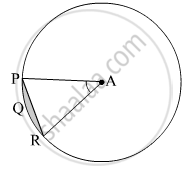Advertisement Remove all ads

# In the Given Figure, If a is the Centre of the Circle. ∠ Par = 30°, Ap = 7.5, Find the Area of the Segment Pqr ( π = 3.14) - Geometry

Advertisement Remove all ads
Advertisement Remove all ads
Advertisement Remove all ads
Sum

In the given figure, if A is the centre of the circle. $\angle$ PAR = 30°, AP = 7.5, find the area of the segment PQR ( $\pi$  = 3.14)Advertisement Remove all ads

#### Solution

Radius of the circle, r = 7.5 cm
∠​PAR = θ = 30º
∴ Area of segment PQR

$= r^2 \left( \frac{\pi\theta}{360°} - \frac{\sin\theta}{2} \right)$
$= \left( 7 . 5 \right)^2 \left( \frac{3 . 14 \times 30° }{360° } - \frac{\sin30° }{2} \right)$
$= 56 . 25 \times \left( \frac{3 . 14}{12} - \frac{1}{4} \right)$
$= 56 . 25 \times 0 . 01167$
$= 0 . 65625 {cm}^2$

Thus, the area of the segment PQR is 0.65625 cm2.

Concept: Areas of Sector and Segment of a Circle
Is there an error in this question or solution?

#### APPEARS IN

Balbharati Mathematics 2 Geometry 10th Standard SSC Maharashtra State Board
Chapter 7 Mensuration
Practice set 7.4 | Q 3 | Page 160
Advertisement Remove all ads
Share
Notifications

View all notifications

Forgot password?